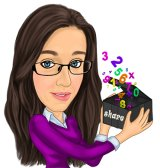0
Shares

Data Worksheets

I have developed my own style of differentiated maths worksheets for lessons which are inspired by the PRET Homework’s. These maths worksheets on algebra are split into several sections. Sometimes it will be relevant for these maths resources to be used over multiple lessons.

Memory – The memory boxes are there to provide students with memory aids or examples. The memory box does not mean necessarily this is the way to start teaching a topic. The maths memory tools support the great teaching within a lesson. Feel free to ignore this box also.

Literacy – Each maths worksheet includes a literacy section. Here students will be asked to recognise key maths words and define them. Alternatively students may be asked to describe a mathematical process. There has been a lot of research carried out which suggests those students who are able to talk about and explain the maths processes often understand and remember larger proportions of the work.

ROK – This stands for Retention of Knowledge. This is a chance for a student to recall their prior maths learning. The maths skills questioned in the retention section give students a chance to practise maths skills they will need to access the lesson. This helps build in a little bit of spaced learning.

Skills – The maths skill sections of the worksheet focus in on the developing new concepts taught on a maths topic. The maths questions often increase in difficulty throughout a skill section. These focus on the maths GCSE exam AO1 calculation skills.

Stretch – These are maths questions designed to stretch and challenge a student. These challenging maths questions interleave topics from other mathematical topics. Stretch questions focus on a student’s ability to mathematically reason (AO2) and problem solve (AO3). These allow students to explore the breadth and depth of a maths topic.

Related Articles@DIRT_expert recently introduced to PRET style maths homework's. PRET stands for Practise, Recall, Extend and Think. The... Data HandlingThese maths resources include bronze, silver and gold maths tasks for the end of a module or revision. They are ideal fo... Data Handling